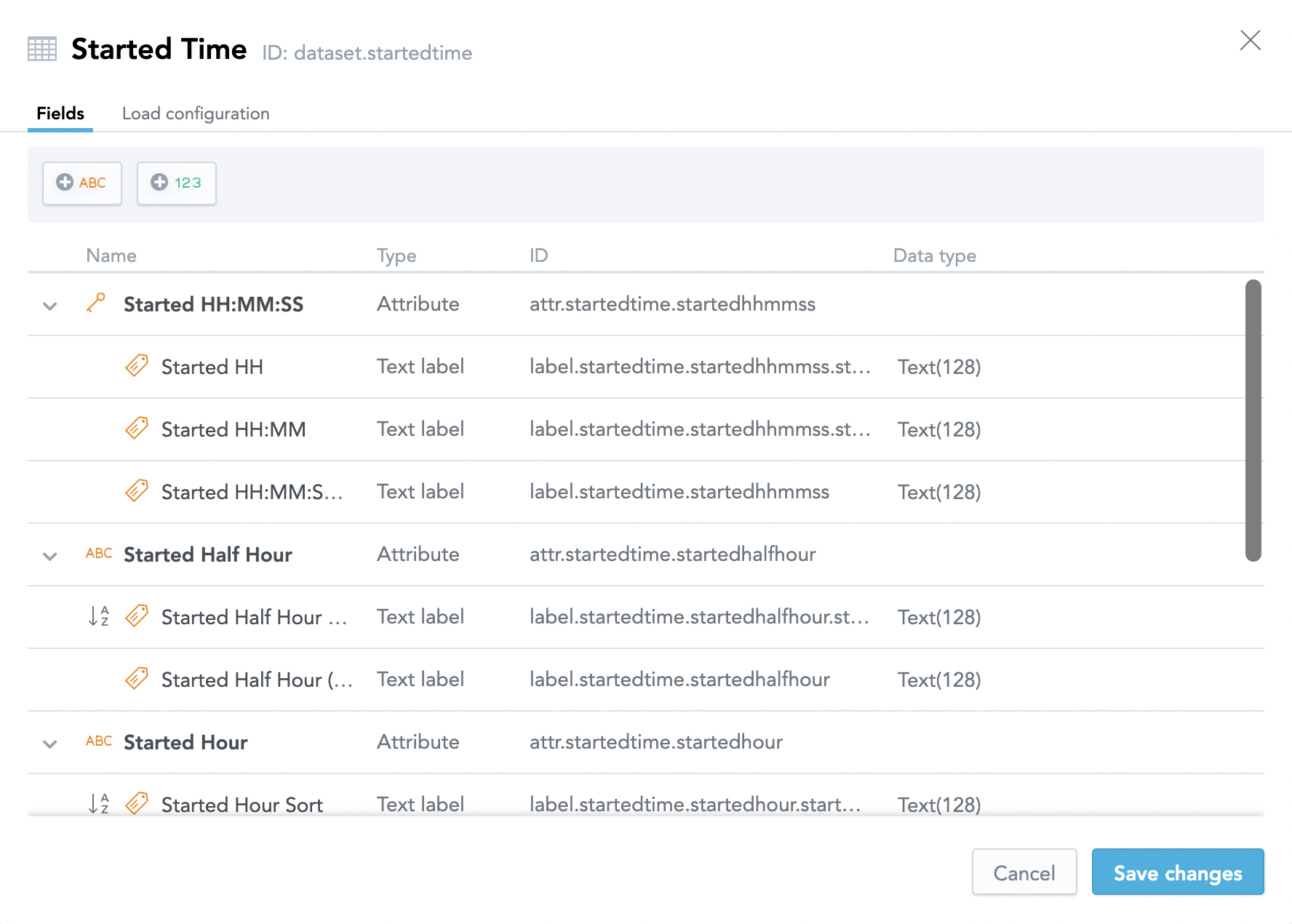# Using a Custom Time Dimension in a Logical Data Model

In some situations, you need to slice your data by timestamps. For example:

• To predict the number of call center personnel required at different times of a day, you may want to see data from a call center sliced by hour and minute or by hour and quarter hour (15-minute intervals).
• To plan the movie schedule at a cinema, you may need to know how many tickets are sold for particular movies at different times of day across a selected range of dates.

To handle such situations, we recommend that you split the date and time into separate attributes. To do so:

1. Create a date dimension for the date attribute using either a standard (default) calendar, or a custom fiscal calendar.
2. Add a custom time dimension to handle the time of day.

You can use the custom time dimension together with a GoodData date dimension for slicing the data, or you can use it independently if you need to see trends by time-of-day across dates.

When you are creating a custom time dimension, consider the following:

• Adding attributes for any custom intervals (for example, quarter hour)
• Adding labels for any custom display formats (for example, 12-hour clock vs. 24-hour clock)
• Adding labels for sorting so that hours, minutes, and seconds are displayed without leading zeros, and so that AM times precede PM times when using a 12-hour clock

## Custom Time Dimensions in a Logical Data Model

The following picture shows a sample logical data model (LDM) for collecting data from a call center. The LDM has two custom time dimension datasets: `Started Time` and `Ended Time`.The following picture shows the attributes and labels in the `Started Time` time dimension dataset:1. Create a table (`wh_time_of_day`) in your Data Warehouse instance using the `TIME` data type.

``````DROP TABLE IF EXISTS wh_time_of_day;
CREATE TABLE wh_time_of_day
(time_of_day        TIME)
SEGMENTED BY HASH(0) ALL NODES
;
``````
2. Generate 24 hours of data:

1. Create a temporary table (`tmp_hour`) for hours with 24 rows containing a single column that holds values of ‘00’ through ‘23’.

``````CREATE LOCAL TEMPORARY TABLE tmp_hour
(hour_of_day     CHAR(2) )
ON COMMIT PRESERVE ROWS;

INSERT INTO tmp_hour VALUES('00');
...
INSERT INTO tmp_hour VALUES('23');
``````
2. Create a temporary table (`tmp_min_sec`) for minutes and seconds with 60 rows containing a single column that holds values of ‘00’ through ‘59’.

``````CREATE LOCAL TEMPORARY TABLE tmp_min_sec
(min_sec_of_day  CHAR(2) )
ON COMMIT PRESERVE ROWS;
INSERT INTO tmp_min_sec VALUES('00');
...
INSERT INTO tmp_min_sec VALUES('59');
``````
3. Load the data to the Data Warehouse table (`wh_time_of_day`). In this example, you are going to truncate and load the `wh_time_of_day` table using `INSERT` with a `SELECT` of all rows from `tmp_hour` with a Cartesian join (`CROSS JOIN`) to `tmp_min_sec` for minutes. The Cartesian join will join each hour (00-23) with minutes (00-59). If the time dimension needs to be down to the second, add another Cartesian join that will join each hour and minute to each second (00-59).

``````TRUNCATE TABLE wh_time_of_day;
INSERT /*+direct*/ INTO wh_time_of_day
SELECT TO_TIMESTAMP(a.hour_of_day || ':' || b.min_sec_of_day || ':' ||
c.min_sec_of_day, 'HH:MI:SS')
FROM tmp_hour a
CROSS JOIN tmp_min_sec b
CROSS JOIN tmp_min_sec c
ORDER BY a.hour_of_day, b.min_sec_of_day, c.min_sec_of_day;
``````
4. Load the output stage table (or GoodData dataset) from the Data Warehouse table (`wh_time_of_day`), using SQL for formatting the desired attributes and labels. The following are some examples of formatting the various attributes and labels:

``````-- SELECT from wh_time_of_day...
-- 24 hour clock time formats
TO_CHAR(time_of_day, 'HH:MI:SS') AS time_hhmmss,
TO_CHAR(time_of_day, 'HH:MI') AS time_hhmm,
TO_CHAR(time_of_day, 'HH') AS time_hh,
-- 12 hour clock time in hours
CASE
WHEN LEFT(TO_CHAR(time_of_day, 'HH12'), 1) = '0'
THEN RIGHT(TO_CHAR(time_of_day, 'HH12 AM'), 4)
ELSE TO_CHAR(time_of_day, 'HH12 AM')
END
AS time_hour,
EXTRACT(HOUR FROM time_of_day) AS time_hour_sort, -- integer for hour sort
``````
``````-- 12 hour clock down to minutes
CASE
WHEN LEFT(TO_CHAR(time_of_day, 'HH12'), 1) = '0'
THEN RIGHT(TO_CHAR(time_of_day, 'HH12:MI AM'), 7)
ELSE TO_CHAR(time_of_day, 'HH12:MI AM')
END
AS time_minute,
(EXTRACT(HOUR FROM time_of_day) * 100) + EXTRACT(MINUTE FROM time_of_day)
AS time_minute_sort,  -- integer HHMM for sorting
``````
``````-- 12 hour clock down to seconds
CASE
WHEN LEFT(TO_CHAR(time_of_day, 'HH12'), 1) = '0'
THEN RIGHT(TO_CHAR(time_of_day, 'HH12:MI:SS AM'), 10)
ELSE TO_CHAR(time_of_day, 'HH12:MI:SS AM')
END
AS time_second,
(EXTRACT(HOUR FROM time_of_day) * 10000) +
(EXTRACT(MINUTE FROM time_of_day) * 100) +
CAST(TO_CHAR(time_of_day, 'SS') AS INTEGER)
AS time_second_sort,  -- integer HHMMSS for sorting
``````
``````-- half hour intervals (similar for quarter hour intervals)
CASE
WHEN EXTRACT(MINUTE FROM time_of_day) < 30
AND LEFT(TO_CHAR(time_of_day, 'HH12'), 1) = '0'
THEN RIGHT(TO_CHAR(time_of_day, 'HH12'), 1) || ':00 ' ||
TO_CHAR(time_of_day, 'AM')
WHEN EXTRACT(MINUTE FROM time_of_day) < 30
THEN TO_CHAR(time_of_day, 'HH12') || ':00 ' ||
TO_CHAR(time_of_day, 'AM')
WHEN EXTRACT(MINUTE FROM time_of_day) < 60
AND LEFT(TO_CHAR(time_of_day, 'HH12'), 1) = '0'
THEN RIGHT(TO_CHAR(time_of_day, 'HH12'), 1) || ':30 ' ||
TO_CHAR(time_of_day, 'AM')
ELSE TO_CHAR(time_of_day, 'HH12') || ':30 ' || TO_CHAR(time_of_day, 'AM')
END
AS time_halfhour,
-- half hour interval sort (integer formatted as HH00 or HH30)
CASE
WHEN EXTRACT(MINUTE FROM time_of_day) < 30
THEN (EXTRACT(HOUR FROM time_of_day) * 100)
ELSE (EXTRACT(HOUR FROM time_of_day) * 100) + 30
END
AS time_halfhour_sort
``````